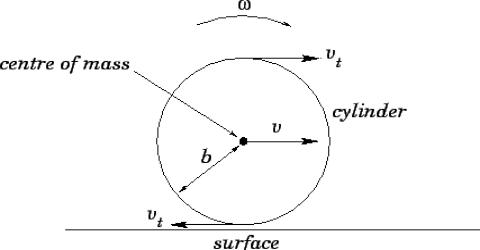# Translational Motion

Translational Motion

Translational motion is the movement of an object from one point to another through space. An example of this is a bullet fired from a rifle.

Suppose, a box is being pushed on the floor to take it somewhere. The box has been shifted from A to B and the point C of the lower portion of the box has been moved to point D.

All the points of the box have been displaced in the same direction travelling the same distance CD. This is an example of motion.

Hence, translational motion is the motion, when an object moves in such a way that all the particles or points of the object traverse the same distance in the same direction taking the same time. Therefore, you are in motion along with the word that means you are moving.

Experiment

Task: Collect one brick. Keep the brick at a definite point A on the table in your class-room. Mark the point i of the front and back edge of the brick by drawing line on the table with a chalk. Now, push the object in a forward direction and take it to a definite distance. Measure the distance between the points of front edge marked on the table to the new position of the point of front edge and also the distance between marked (point and the new position of back edge point.

You will find both the distances the same. Similarly, it you measure the distance of the middle point to the new middle point you will find the same distance.

The motion of the drawer of a table the motion of a box sliding along an inclined plane and the motion of the hand while writing- all of these are translational motions. Translational motion may be divided into two kinds:

(1) Rectilinear motion and

(2) Curvilinear motion.

When we write, our hand travels in a straight line for some times and again through different curve lines in other times. When an object travels in a straight line, it is called rectilinear motion. Again when an objects travels through curved line, the motion is called curvilinear.